# Geometric Decompositions

A remarkable theorem involving decompositions is that if one has two plane simple polygons of the same area, it is possible to decompose either of the polygons into polygonal pieces that can be reassembled to form the other polygon…

# Geometric Decompositions

Joe Malkevitch
York College (CUNY)

### Introduction

When looking at a body of mathematical ideas, one might look for the “atoms” or parts so that one could see the whole by having insight into its parts. If in some future state of the Earth there were no automobiles and some humans came across a well preserved car from the 1970’s, but with no prior knowledge of what such a thing was, how might they interpret what they were looking at? An archaeologist at that time might try to understand its parts as a way to think through what the whole thing was good for. Perhaps these people might decide it was a small moveable house?

In an earlier Feature Column essay I looked at how by studying primes 2, 3, 5, 7, … we get insight into big integers such as 1111113. There I also looked at partitions of positive integers—for example, $5 = 4 + 1$ and $5 = 3 + 1 + 1$ are but two of the partitions of 5. Words connoting or related to decomposition in English are: decompositions, dissections, factoring, irreducible, etc. It is not uncommon in mathematics to use words as technical vocabulary that suggest ideas that a word has in more ordinary usage, that is non-mathematical contexts. For example, consider the word "irreducible". This suggests something that cannot be broken up into parts.

### Geometric Decomposition

Before addressing the issue of geometric compositions in earnest, as a teaser remember that one of the most important and well known theorem in mathematics is the Pythagorean Theorem, though attributing it to Pythagorus or even the Pythagoreans distorts the history of this remarkable result, which can be viewed as a result in algebra or a result in geometry. The Pythagorean Theorem states that if one has a right triangle (one where two sides meet at a 90 degree angle—that is, are perpendicular), the square (in the algebraic) sense of the lengths of the side opposite the right angle is the sum of the squares of the lengths of the other two sides. But this theorem about lengths can also be interpreted as a statement about the areas of the geometric squares that can be constructed on the sides of a right triangle. Here are diagrams (Figures 1 and 2) that support one of the many proofs of the Pythagorean Theorem that involved moving around pieces of squares and assembling them to form other squares.Figure 1 (A proof of the Pythagorean Theorem based on decomposing the squares into pieces that can be reassembled in other ways. Diagram courtesy of Wikipedia.)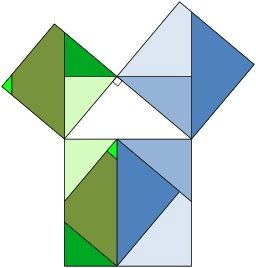Figure 2 (A proof of the Pythagorean Theorem based on reassembling the pieces of squares on the sides of a right triangle, shown in white. Image courtesy of Wikipedia.)

Proofs of theorems using "physical models" such as the diagrams in Figures 1 and 2 are quite compelling because of the amazing ability of humans to input and process visual information. The eye responds to issues related to the length of segments and the area of regions, even if sometimes the fact that area scales as the square of length rather than length itself sometimes causes individuals to make misleading judgments about diagrams.

One might try to understand geometric objects in terms of the parts that make them up. These parts might be described as: points, lines, membrane patterns, corners, curves, etc. Sometimes these parts can be viewed with terms that overlap. In describing a shirt one might use terms like sleeves and buttons and in describing a car one might mention windows and wheels. Here we will give special consideration to the notion of a polygon.

After the point and the line, among the most fundamental of geometrical objects is the triangle. A triangle is a collection of three points not on a line and the segments joining pairs of the points which are known as the vertices of the triangle. When we classify polygons that are drawn on a flat piece of paper in the plane, we can do so by counting the number of corners of the polygon or by counting the number of sides (edges) of the polygon. We can think of a polygon as a collections of points joined by sticks with no membrane filling in the result or we can include the interior of the polygon along with the "sticks." There are pros and cons for defining shapes in particular ways. Here I just want to point out that we can classify polygons drawn in the plane, not only by their number of corners, but by whether or not the polygon intersects itself or has notches. We say a set $X$ is convex if given any two elements $u$ and $v$ of $X$ the line segment joining $u$ and $v$ is contained in (is a subset of) $X$.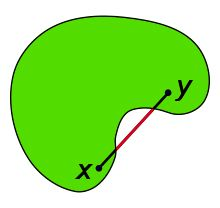Figure 3 (A diagram illustrating the idea of convexity. Courtesy of Wikipedia.)

Figure 3 provides an illustration of this fundamental concept of modern geometry. Intuitively, a convex set is one that does not have notches or holes. In particular polygons drawn in the plane are usually defined (as are circles) to be dots connected by straight line segments—"sticks"—without the points in the interior. Triangles with their interiors are convex sets but as soon as we have a polygon with more than 3 vertices we can have non-convex polygons, polygons whose vertices do not lie in a plane, or polygons whose sides self-intersect. In some situations polygons are allowed to have several consecutive vertices lying along a straight line, but often it is required that pairs of consecutive vertices not lie along the same line. This polygon has 6 vertices (corners) and 6 sides, and is thus often described as a hexagon, in this case, a non-convex hexagon.

Historically, attention has been given to the length of sides and the measure of the interior (and sometimes exterior) angles of polygons. When the angles of a convex polygon are equal and its sides have the same length, it is called regular. However, one can consider polygons where the sides are all equal, that is the polygon is equilateral, or the angles are all equal, in which case it is equiangular. The polygon in Figure 4 is an equilateral hexagon. Its angles are not all equal, but there are three different sizes of angle, equal in pairs. If one adopts a partition-style way of classifying this polygon it is an example of a non-convex $\{6\}$; $\{2, 2, 2\}$ since there are six sides of equal length, and three types of angles equal in pairs.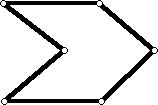Figure 4 (A non-convex hexagon where all of the sides have equal length.)

Figure 5 shows a small sampler of polygons, one convex and others non-convex. In one case, all consecutive pairs of sides of the polygon meet at right angles (a rectilinear or orthogonal polygon).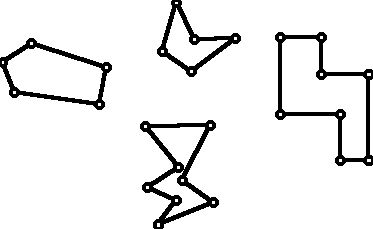Figure 5 (A sampler of different kinds of polygons, convex and non-convex.)

The simplest polygon is one that has only three vertices, a triangle. In the spirit of decomposition, it is natural to ask if every plane simple polygon can be decomposed using existing vertices into triangles. While this may seem intuitively obvious, it is actually not that easy to prove this fact, though it is true. It may seem intuitively clear that the 3-dimensional analogues of polyhedra, including ones that are non-convex but have the topology of a sphere can be decomposed into tetrahedra (e.g. the "atoms" of 3-dimensional convex polyhedra, as it were), but this is in fact not true!

Given a polygon with vertices drawn in the plane, it is always possible to subdivide that polygon into triangles using existing vertices. However, for some decomposition problems it is of interest to add additional vertices to the sides of the polygon as part of the decomposition effort, and sometimes one might also allow having vertices in the interior of the polygon. Thus in Figure 12 you can see how a square can be subdivided into triangles by adding some additional vertices along the side of the square and also how to do the subdivision using an additional vertex. Figure 6 shows a simple (no self-intersections) non-convex polygon with 11 vertices (11 sides). Using 8 segments joining existing vertices this 11-gon can be subdivided into triangles using 8 diagonals and giving rise to 9 triangles. In fact there are many other such triangles starting with this same polygon but all of them will involve using 8 diagonals and give rise to 9 triangles. In general any simple $n$-gon ($n$ at least 4) can be triangulated using
$(n-3)$ diagonals into $(n-2)$ triangles. (One can see this using Euler’s Polyhedral Formula for a connected graph: $V + F – E = 2$ for a connected graph drawn in the plane.)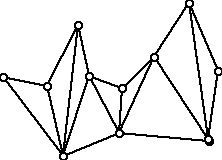Figure 6 (A simple non-convex polygon converted using diagonals to a polygon subdivided into triangles.)

Figure 7 and Figure 8 show (Figure 7) a convex 9-gon subdivided by 6 diagonals into 7 triangles and (Figure 8) a non-convex 9-gon subdivided by 6 diagonals into 7 triangles. Unlike the triangulation in Figure 6, each of these triangulations includes one (or more) triangles which share edges with three other triangles, something which does not occur for Figure 6.Figure 7 (A convex polygon subdivided into triangles.)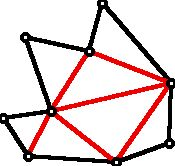Figure 8 (A non-convex polygon partitioned into triangles using the diagonal edges shown in red.)

In Figure 8 I have called attention to the edges that subdivide the original polygon into triangles by coloring the subdividing diagonals red. As a problem in graph theory (the theory of diagrams involving dots and the lines that join them), you may want to think about the question:

Given a collection of edges, when can they serve as the diagonals for a plane $n$-gon that turns the $n$-gon into a triangulated polygon?

A remarkable theorem involving decompositions is that if one has two plane simple polygons of the same area, it is possible to decompose either of the polygons into polygonal pieces that can be reassembled to form the other polygon. This result is known as the Wallace-Bolyai-Gerwien Theorem. By way of illustration, Figure 9 shows a way to decompose a square and equilateral triangle of equal area into polygonal parts that can be used to form the other shape. The decomposition shown uses the minimal number of pieces. A lot of research has been done on equidecomposibility with a minimal number of pieces and where one uses particular shapes for the pieces in the decomposition.Figure 9 (A square and equilateral triangle of the same area can be decomposed into 4 pieces which can be assembled to form the other shape. Image courtesy of Wikipedia.)

It is natural to ask if, in 3 dimensions, two polyhedra with the same volume can have one be decomposed (cut) into pieces and reassembled to form the other. This problem was solved by Max Dehn (1876-1952) and was one of a set of famous problems designed by David Hilbert (1862-1943), whose solution he thought would create progress in a variety of mathematical areas. Figure 10 shows two 3-dimensional polyhedra, one decomposed into the other. Dehn provided tools for telling when this could be done. However, it may surprise you to learn that the analogue of what we see in Figure 9 can’t be achieved, that is, a regular 3-cube (see left of Figure 10) can’t be cut into polyhedral pieces and reassembled to a regular tetrahedron of the same volume.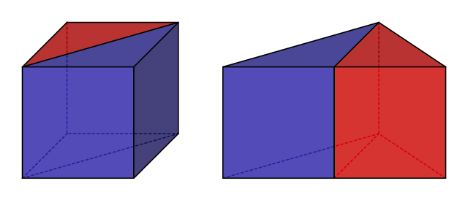Figure 10 (A cube decomposed into a triangular prism of the same volume. Image courtesy of Wikipedia.)

### Decomposing Squares

A natural question about decomposition of a polygon is whether or not the polygon can be decomposed into convex pieces, and in particular triangles, which will automatically be convex, where the pieces can be made to have equal area.

Each of the squares in Figure 11 can be thought of as squares of side length 2 and both have been divided into 4 congruent triangles, and thus, for the one on the left the 4 triangles have the same area and the same is true on the right. It is interesting that in each case the triangles are special because they are right triangles and thus satisfy the Pythagorean theorem. You can verify for yourself that on the left, the right triangles are isosceles right triangles with sides $\sqrt{2}$, $\sqrt{2}$, and 2 while on the right the triangles are scalene (all three sides of different lengths) and these lengths are 1, 2, $\sqrt{5}$. Also note that although the squares above are meant to be congruent, both of side length 2, it may not appear that the smaller triangles on each side have equal area but you can verify using the Pythagorean Theorem that the small triangles on the left have the same area as the smaller triangles on the right.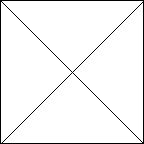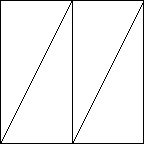Figure 11 (Two different ways to subdivide congruent initial squares into 4 congruent triangles.)

The decompositions of squares into equal area triangular parts in Figure 12 can be extended to decomposing a square in various ways into an even number of parts. The decompositions shown in Figure 11 have the property that when two triangles touch each other they touch along a complete edge of another triangle. Are other kinds of decompositions of a square into an even number of triangles possible? Figure 12 shows an example of how a square can be decomposed into 6 parts, and not all of the triangles are right triangles, where some of the triangles meet other triangles along a part of an edge rather than a full edge, and yet the 6 parts can be shown to have equal area. Triangles which touch in this way are said not to meet edge-to-edge. In recent years interest in tilings of the plane as well as polygons into pieces with special properties allows for tiles that don’t meet edge-to-edge.Figure 12 (A decomposition of a square into 6 triangles of equal area, where not all of the triangles meet edge-to-edge. Image courtesy of Wikipedia.)

What almost certainly has already occurred to you regarding this discussion is decomposing a square into an odd number of triangles with the same area. If you try to find such a dissection you will find that you are not able to do this! So you might try to prove that it cannot occur. However, if you are like many people you will not find it so easy to do this. Recently, the following theorem has been associated with the name Paul Monsky:

Theorem (1970): It is not possible to decompose a square into an odd number of triangles of equal area.

Like many easy-to-state questions that are not so easy to demonstrate there is a lot of history in how Monsky came to show his result. It might seem that the history would go back into ancient times but in fact the problem seems to have been born quite recently. The origin of the problem appears to have occurred in 1965 with Fred Richmond and other names associated with the problem are John Thomas and Sherman Stein. While many saw the problem with the publication of Monsky’s proof in 1970, it was work of Sherman Stein that magnified interest in the problem under the title of what have come to be called equidissection problems. What Stein basically did was to ask what other shapes in the plane were such that they could not be dissected into triangles of equal area. You might want to think about this issue for yourself and perhaps you can come up with some new variants that other people have not thought of. After all why restrict oneself to squares!

The fact that a square can’t be decomposed into an odd number of squares of the same area does not mean that one can’t think about dividing squares into an odd number of triangles such as those in Figure 13. We know that the
triangles in such a decomposition cannot have equal area, but researchers have investigated the issue of how close to being equal in area they can be made in terms of the number of triangles in the decomposition.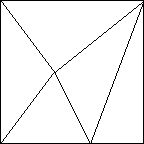Figure 13 (A square decomposed into an odd number of triangles. Such a decomposition with all the triangles having equal area is not possible.)

Much more recent than the ideas that lead to Monsky’s Theorem has been the idea of investigating when one can take a convex polygonal region in the plane and decompose it into $N$ convex pieces which have the same area and perimeter! A published version of this challenge appeared in a paper posted to the ArXiv in 2008 by R. Nandakumar and N. Ramana Rao which has subsequently, in the spirit of Monsky’s Theorem, attracted additional interest in this question and its generalizations.
This problem, while very easy to state, has inspired a huge amount of new geometrical facts as well as many new questions. It is very common in the process of making progress on one mathematical problem that it opens up new questions, the need to invent new tools and sometimes whole new clusters of mathematical questions.

We have looked at how surprisingly rich and complex the environment of decomposing a polygon into triangles can be and, in particular, the decomposition of a square into triangles of equal area. What about decomposing a square into squares subject to various rules? Clearly one can take a square and decompose it into various numbers of other squares of the same area, and the number of squares in the decomposition can be even or odd. However, much earlier than the interest in what has come to be called Monsky’s Theorem, a group of British students, all of whom went on to distinction in various ways, R.L. Brooks, Cedric Smith, Arthur Stone and William Tutte, while students at Cambridge University in the 1930s looked at a problem which has lead to much important and interesting work in various parts of mathematics and is, again, very much a decomposition theorem. The idea is to take a square (or once generalized as a question, rectangle) and divide it into squares with the initially curious restriction that all of the squares in the decomposition have different side lengths. This problem has come to be known as the perfect square problem. Figure 14 shows an interesting example that fails to achieve this goal but is nonetheless striking for what it does accomplish.Figure 14 (A square of relatively small side length subdivided into smaller squares some of which have the same edge length. Image courtesy of Wikipedia.)

Figure 15 shows an example of a square with the property that all the subdividing squares have different edge lengths.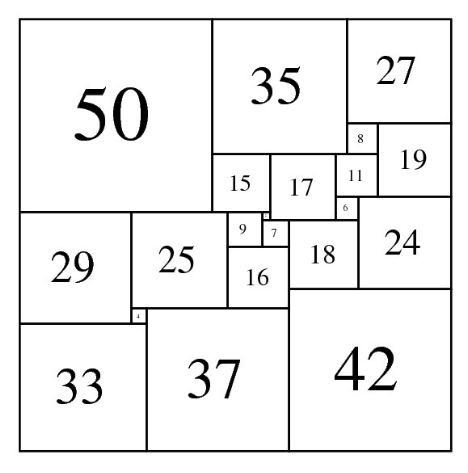Figure 15 (A square subdivided into squares all of which have different edge lengths. Image courtesy of Wikipedia.)

There are many "windows" (some not square) that serve as entries into mathematical insights and investigations. Looking at parts or decompositions of shapes as well as numbers leads to lots of fascinating mathematics and its applications.

References

Those who can access JSTOR can find some of the papers mentioned above there. For those with access, the American Mathematical Society’s MathSciNet can be used to get additional bibliographic information and reviews of some of these materials. Some of the items above can be found via the ACM Digital Library, which also provides bibliographic services.

Abrams, Aaron, and Jamie Pommersheim. "Generalized dissections and Monsky’s theorem." Discrete & Computational Geometry (2022): 1-37.

Alsina, Claudi, and Roger B. Nelsen. Math made visual: creating images for understanding mathematics. Vol. 28. American Mathematical Soc., 2006.

Akopyan, Arseniy, Sergey Avvakumov, and Roman Karasev. "Convex fair partitions into an arbitrary number of pieces." arXiv preprint arXiv:1804.03057 (2018).

Alsina, Claudi, and Roger B. Nelsen. A Cornucopia of quadrilaterals. Vol. 55. American Mathematical Soc., 2020.

Frederickson, G. Dissections: Plane and Fancy. New York: Cambridge University Press, pp. 28-29, 1997.

Hoehn, Larry. A New Proof of the Pythagorean Theorem: Mathematics Teacher. February 1995; NCTM: Reston, VA.

Jepsen, Charles, and Roc Yang. "Making Squares from Pythagorean Triangles." The College Mathematics Journal 29.4 (1998): 284-288.

Karasev, Roman, Alfredo Hubard, and Boris Aronov. "Convex equipartitions: the spicy chicken theorem." Geometriae Dedicata 170.1 (2014): 263-279.

Kasimatis, Elaine Ann. "Dissections of regular polygons into triangles of equal areas." Discrete & Computational Geometry 4.4 (1989): 375-381.

Katz, Victor J. . A History of Mathematics. 1993; Harper Collins: New York, New York.

Loomis, E. S. The Pythagorean Proposition: Its Demonstrations Analyzed and Classified and Bibliography of Sources for Data of the Four Kinds of "Proofs," 2nd ed. Reston, VA: National Council of Teachers of Mathematics, 1968.

Machover, M. "Euler’s Theorem Implies the Pythagorean Proposition." Amer. Math. Monthly 103, 351, 1996.

Maldonado, Gerardo L., and Edgardo Roldán-Pensado. "Dissecting the square into seven or nine congruent parts." Discrete Mathematics 345.5 (2022): 112800.

Maor, Eli. The Pythagorean theorem: a 4,000-year history. Vol. 65. Princeton University Press, 2019.

Mead, David G. "Dissection of the hypercube into simplexes." Proceedings of the American Mathematical Society 76.2 (1979): 302-304.

Monsky, Paul. "On dividing a square into triangles." The American Mathematical Monthly 77.2 (1970): 161-164.

Nelsen, Roger B. Proofs without words: Exercises in visual thinking. No. 1. MAA, 1993.

Posamentier, Alfred S. The Pythagorean theorem: the story of its power and beauty. Prometheus books, 2010.

Nandakumar, R., and N. Ramana Rao. "Fair partitions of polygons: An elementary introduction." Proceedings-Mathematical Sciences 122.3 (2012): 459-467.

Rooney, Elaine Ann Kasimatis. "DISSECTION OF REGULAR POLYGONS INTO TRIANGLES OF EQUAL AREAS." (1987): 4188-4188.

Stein, Sherman. "Equidissections of centrally symmetric octagons." Aequationes Mathematicae 37.2 (1989): 313-318.

Stein, Sherman K. "Cutting a polyomino into triangles of equal areas." The American Mathematical Monthly 106.3 (1999): 255-257.

Stein, Sherman. "A generalized conjecture about cutting a polygon into triangles of equal areas." Discrete & Computational Geometry 24.1 (2000): 141-145.

Stein, Sherman. "Cutting a polygon into triangles of equal areas." The Mathematical Intelligencer 26.1 (2004): 17-21.

Su, Zhanjun, and Ren Ding. "Dissections of polygons into triangles of equal areas." Journal of Applied Mathematics and Computing 13.1 (2003): 29-36.

Wang, Yang, Lei Ren, and Hui Rao. "Dissecting a square into congruent polygons." Discrete Mathematics & Theoretical Computer Science 22 (2020).